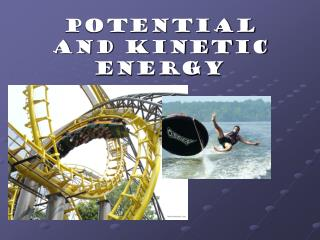DownloadDownload PresentationPotential and Kinetic Energy

# Potential and Kinetic Energy

Download Presentation## Potential and Kinetic Energy

- - - - - - - - - - - - - - - - - - - - - - - - - - - E N D - - - - - - - - - - - - - - - - - - - - - - - - - - -
##### Presentation Transcript

1. Potential and Kinetic Energy

2. Work = force x distance (W=Fd) When work occurs 2 things happen: The application of a force The movement of something by that force The unit of measurement is a combination of force (N) with distance (m) to give a newton-meter (N-m) or the Joule (J). Rhymes with cool.

3. 2 loads lifted one story 1 load lifted two stories Both illustrations above show someone doing twice as much work. The first has twice the force required to lift 2 loads. The second has twice the distanceto lift one load. The illustration below shows a weight lifter holding up a barbell, doing no work. You do work on something when you force it to move against the influence of an opposing force – often friction. No Work = No Distance

4. How FAST the work is done is called Power. Power = work done / time Twice the power means an engine can do twice the work in the same amount of time or the same amount of work in half the time. The unit for is the joule per second, also known as the watt. In the U.S. we usually rate engines in units ofhorsepower & electricity in units of kilowatts. In The metric system, autos are rated in kilowatts.

5. The three main engines of the space shuttle can develop 33,000 MW (mega- watts) of power when fuel is burned at the enormous rate of 3,400 kg/s. This is like emptying an average- size swimming pool in 20 seconds!

6. The “something” that enables an object to do work is Energy. The two most common forms of fall under the category of mechanical energy. Mechanical Energy = the energy due to the position of something, or the movement of something.

7. Kinetic Energy Potential Energy Elastic Potential Energy Gravitation Potential Energy Chemical Potential Energy How is all energy divided? Mechanical Energy

8. What is Potential Energy? • Energy that is stored and waiting to be used later

9. What is Gravitational Potential Energy? • Potential energy due to an object’s position • P.E. = mass x height x gravity Don’t look down, Rover! Good boy!

10. What is Elastic Potential Energy? • Potential energy due compression or expansion of an elastic object. Notice the ball compressing and expanding

11. What is Chemical Potential Energy? • Potential energy stored within the chemical bonds of an object

12. What is Kinetic Energy? • Energy an object has due to its motion • K.E. = .5(mass x speed2)